# The Model Student: A Game of Six Integers (Part 1)

The Model Student: A Game of Six Integers (Part 1)

By Richard Harris

In how many ways can you combine a set of numbers? Richard Harris gets counting.

Dum de dum de dum, dum de dum de dum, dum de dum de dum, dum de dum de dum, baa daa daa, baa daa daa, boo doo, boo doo, boo doo dee doo, choo.

Ah, Countdown. A nice cup of tea, perhaps a choccy biccy or two, and we're ready to cross mental swords with televisual gladiators in half an hour of orthographical and arithmetical battle.

Er, sorry, it seems I've set the hyperbole switch to turbo.

Hang on a sec.

Righty ho.

Beloved of students, retirees and housewi..., er, home-makers throughout the land, it is one of the longest running game shows in the world and was the very first program broadcast on Channel 4, way back in 1982 [ Countdown ].

Hosted for much of its astonishingly long run by Carol Vorderman and the splendidly be-blazered and much missed Richard Whiteley, we have entered a new era with the recent departure of the former and the premature demise of the latter.

For the one or two of you who haven't experienced the joy of Countdown, the game consists of two types of rounds; the letters rounds and the numbers rounds.

In the letters rounds, one of the two contestants chooses 9 letters from a random source of consonants and a random source of vowels with which they both seek to construct as long a word as possible.

In the numbers rounds, one of the contestants chooses 6 integers from a concealed set of large integers and a concealed set of small integers with which they both seek an arithmetic formula that yields a value as close as possible to a randomly selected target between 1 and 999.

Guess which one I'm interested in? You got it.

## The Countdown numbers game

Before the start of the numbers game the two sets of large and small numbers, printed on cards, are randomly shuffled and placed face down upon a table.

Specifically, the set of large numbers, consisting of one of each of 25, 50, 75 and 100, are placed upon the table above the set of small numbers, consisting of two of each of the integers from 1 to 10, so as to distinguish between them.

One of the contestants then picks 6 of these, typically 1 large and 5 small, before a random target between 100 and 999 is generated.

Using addition, subtraction, multiplication and division they both must then, during a period of just 30 seconds, attempt to discover a formula that yields the target number using each of these numbers no more than once. Furthermore, every intermediate value during the calculation, and hence the result itself, must be a whole number. For example, if the selected numbers were 75, 8, 6, 5, 3, 3 and the target were 234, we would hit it exactly with the formula:

75 × 3 + 6 + 3

or, without using the large number, with:

5 × 6 × 8 − 3 − 3

Strictly speaking, a contestant earns points if he or she is the nearest of the pair to the target, provided at least that their result is within a certain maximum difference; let's be honest though, almost is just another word for fail.

Before we can investigate the properties of the numbers game, we shall need some code with which we can enumerate every possible formula that we might construct.

In order to do this, we shall express the formulae in Reverse Polish notation; a spectacularly convenient notation for algorithmically evaluating arithmetic formulae.

## Reverse Polish notation

In Reverse Polish notation, or RPN, arithmetic operators are placed immediately to the right of their arguments rather than between them, as in the more familiar infix notation. For example, the formula 3 + 4 would be expressed as 3 4 + in RPN.

Developed in the late 1950s by Charles Hamblin, it was used to simplify the electronics in early Hewlett-Packard calculators [ HP1 ] and proved so popular that they still support it on some of their models [ HP2 ].

The principal advantages of RPN are that the operators appear in the input sequence precisely when they need to be applied, and that the precedence of the operators is unambiguously determined by their position, eliminating the need for brackets.

We can see this when we express more complex formulae in RPN and to do that we must introduce the RPN stack.

Whenever a number appears in the input sequence of an RPN formula, it is pushed on to the stack. Whenever an operator appears, it pops the arguments it requires off of the stack and pushes the result of the calculation back on to the stack.

An error occurs if there are not enough values on the stack for an operator or if there is more than one value left on the stack at the end of the expression.

For the simple example 3 4 +, we push 3 then 4 on to the stack and the + operator pops them and pushes 7 on to the stack. Since we have reached the end of the expression, this single value on the stack is the result of the calculation.

Using infix notation, we must consider operator precedence to ensure that we arrive at the correct result. For example, the formula

2 + 3 × 4

should be interpreted as

2 + (3 × 4)

rather than

(2 + 3) × 4

since multiplication has higher precedence than addition. If we wish to calculate the latter, we must use brackets to ensure that the operators are applied in the correct order.

In RPN, however, no such complications exist. The first calculation is described by the formula

3 4 × 2 +

and the second by

2 3 + 4 ×

Running through the steps required to calculate the first of these, we push first 3 then 4 onto the stack. The multiplication operator pops these values off of the stack and pushes their product, 12, back on to it. The value 2 is then pushed onto the stack and the addition operator pops off it and the 12 and pushes back their sum, 14. Since there are no more inputs, this is the result of the calculation.

Figure 1 illustrates graphically the state of the stack after each term in the formula is entered.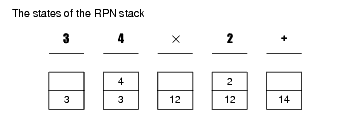Figure 1

## Enumerating the RPN formulae

Noting that the four arithmetic operators allowed by the Countdown numbers game, addition, subtraction, multiplication and division, all require precisely two arguments we need only generate RPN formulae consisting of binary operators.

Rather than generate the formulae directly, I propose that we work with a pair of placeholder symbols, o and x , to represent operators and arguments respectively. We can then subsequently substitute them with every valid permutation of operators and arguments to generate the full set of formulae.

Generating the set of formula templates is a relatively straightforward process. We start with the simplest template, namely a single value x . The next step is to replace the single x with a binary operation and its arguments, xxo , the result of which will also be a single value. Continuing in the same vein, we recursively replace each x in turn in the current template with xxo , stopping when there are as many x symbols as there are available arguments.

For example, if we had 3 arguments to work with, we would traverse the following set of formulae.

x

xxo

xxoxo xxxoo

In general, some formula templates will be generated more than once during the recursion. For example, if we were working with 4 arguments the template xxoxxoo could be generated by replacing with xxo both the last x in xxoxo and the first x in xxxoo from the 3 argument templates. We will therefore need to keep track of the templates we generate in order not to repeat ourselves.

As it happens, it is rather convenient to use strings of x and o characters to represent our formula templates. Listing 1 illustrates the all_templates function that returns the full set of templates for a given number of available arguments.

 ``` std::set all_templates(size_t arguments) { std::set result; if(arguments!=0) all_templates("x", arguments-1, result); return result; } ``` Listing 1

This simply forwards the work on to another overload, provided in listing 2.

 ``` void all_templates(const std::string ¤t, size_t arguments, std::set &result) { result.insert(current); if(arguments!=0) { std::string::size_type pos = current.find('x'); while(pos!=std::string::npos) { std::string next = current; next.replace(pos, 1, "xxo"); if(result.insert(next).second) { all_templates(next, arguments-1, result); } pos = current.find('x', pos+1); } } } ``` Listing 2

The key to terminating the recursion when we have exhausted the set of arguments lies in the fact that each time we replace an x with an xxo we add a single extra argument to the formula template. By passing the remaining number of arguments to the function during the recursion, we can stop when they reach 0.

The main loop iterates over the current template, replacing each x with xxo in turn and passing each new template recursively to the function for the same treatments.

The complete set of formula templates for up to 5 arguments is illustrated in figure 2, in order of increasing length.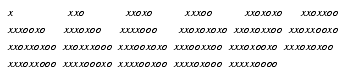Figure 2

## The number of formula templates

One very simple calculation we can perform at this point is to determine the total number of unique formula templates for up to any given number of arguments; we simply call the size member function of the set returned by the all_templates function.

Note that the difference between the result of this calculation for n arguments and the result for n -1 arguments gives us the number of unique formula templates with exactly n arguments.

Table 1 gives the results of both calculations, which we denote by T 1, n and T n respectively, for 0 to 15 arguments.

n T 1,n Tn
0 0 0
1 1 1
2 2 1
3 4 2
4 9 5
5 23 14
6 65 42
7 197 132
n T 1,n Tn
8 626 429
9 2,056 1,430
10 6,918 4,862
11 23,714 16,796
12 82,500 58,786
13 290,512 208,012
14 1,033,412 742,900
15 3,707,852 2,674,440
Table 1

## The number of formulae

From this point it is a relatively simple task to calculate the total number of formulae expressible with a given number of arguments. We shall treat every argument as if it were distinct from all the others since this both dramatically simplifies the calculation and will ultimately yield the correct statistical properties of the Countdown numbers game. Furthermore, we'll restrict ourselves to the 4 binary operators allowed in the numbers game.

Note that we consider formulae distinct even if they could be rearranged to be identical.

To recover the number of formulae with a specific number of arguments, which we shall denote by F n , we must multiply the number of templates by the number of ways we can replace the o symbols and by the number of ways we can replace the x symbols.

Noting that the templates always contain one less operator than the number of arguments, the first multiplier is given by 4 n -1 .

The second multiplier is simply the number of orderings of the n arguments, n factorial or n !, calculated by multiplying together every number from 1 up to and including n . This hold true since when ordering the n arguments we first pick 1 of the n , then 1 of the remaining n -1, then one of the remaining n -2 and so on.

Hence the number of formulae is given by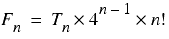The formula for the number of equations with up to a given number of arguments is a little more complex, since in this case we must sum the number of ways we can construct formulae with subsets of the arguments.

The number of ways we can select r from n items when the order of selection is important is known as a permutation and is denoted by n P r .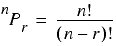This follows in a similar way to the derivation of the number of orderings of n arguments being equal to n !. We first select 1 from the n arguments, then 1 from the remaining n -1 and so on, but this time we stop after we have chosen r of them.

Note that 0! equals 1 and so when r equals n , n P r is simply n !.

The number of formulae with up to n arguments, which we shall denote by F 1, n is therefore given by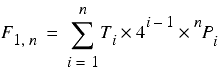Recall that the large capital sigma means the sum of the expression to its right with i taking values from 1 up to and including n .

Table 2 gives the results of these calculations for 0 to 15 arguments.

n F1,n Fn

0

0

0

1

1

1

2

10

8

3

219

192

4

8,500

7,680

5

470,485

430,080

6

33,665,406

30,965,760

7

2,951,054,575

2,724,986,880

8

3.06090E+11

2.83399E+11

9

3.66592E+13

3.40078E+13

10

4.97823E+15

4.62507E+15

11

7.55804E+17

7.03010E+17

12

1.26855E+20

1.18106E+20

13

2.33230E+22

2.17314E+22

14

4.66154E+24

4.34629E+24

15

1.00633E+27

9.38798E+26

Table 2

We can now calculate the total number of formulae that might be expressed during the Countdown numbers game by multiplying F 1,6 by the number of ways we might select the 6 numbers from the 24 on offer. In this case, the order of the number is unimportant and the number is known as a combination, denoted by n C r .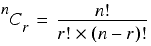This result follows from the fact that the number of combinations is equal to the number of permutations divided by the number of orderings of the r arguments we have chosen.

The total number of possible formulae is therefore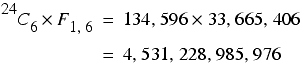So, rather a lot then.

## Is there an explicit formula?

Personally, I'd much rather have an explicit, or closed form, formula for T n than rely upon calling the, as it happens rather computationally expensive, all_templates function over and over again. To this end, I spent some time mucking about with a spreadsheet and came up with, for n greater than or equal to 1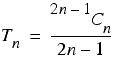This works for every example we've seen so far and, given that the problem is clearly a combinatorial one of some sort or another and that a formula with n arguments has n -1 operators and hence has precisely 2 n -1 terms, it doesn't seem entirely unreasonable.

But, of course, 15 working examples and suspiciously familiar terms are a far cry from an actual proof. Damn you mathematics, you harsh mistress you! Would that you were more like Physics, or better yet Philosophy, so I could get away with any old tosh.

One thing that stands out is that the numerator of the fraction is equal to the number of ways in which one can construct a sequence of n x symbols and n -1 o symbols, since it is the number of ways in which we can pick the n locations we wish to place x symbols. It is a combination, rather than a permutation, since the order in which we pick the locations doesn't matter, just the locations themselves.

This leads to the interpretation that the probability of such a sequence is a valid formula template is equal to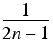To demonstrate that this is indeed the case we first note that such a sequence is a valid formula template if and only if there have been more x symbols than o symbols up to and including each and every term.

If this were not so, we would run out of values on the stack at some point, and hence would not have a valid formula template.

Noting that each operator reduces the number of values on the stack by 1, we must therefore have at the end of the calculation a single value on the stack, representing the result of the formula.

Since it implies that we cannot run out of arguments and that we end the calculation with a single value on the stack, this rule ensures that the formula template is valid.

Thankfully, we don't actually need to prove that this rule is obeyed with the presumed probability, since someone has already done it for us.

## The Ballot Theorem

The Ballot Theorem [ Feller68 ] was proven in 1878 by W. A. Whitworth and can be stated as

Suppose that, in a ballot, candidate P scores p votes and candidate Q scorces q votes, where p>0 and p≥q. The probability that throughout the counting there are always more votes for P than for Q equals (p-q)/(p+q).

Our problem is a special case where P represents the x symbols and receives n votes and Q represents the o symbols and receives n -1 votes. According to the Ballot Theorem, the probability that the number of x symbols exceeds the number of o symbols up to and including each and every term is therefore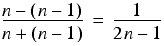as suspected.

There are several ways to prove this theorem [ Renault07 ], but to my mind the most elegant by far uses proof by induction, as shown in derivation 1.

 We first consider the boundary conditions of p equal to q and of p greater than q and q equal to 0. In the first case the number of votes must be equal when we finish counting, so the probability that P always has more votes then Q is 0. The formula correctly predicts this, since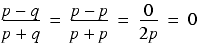In the second case, the number of votes for P must always exceed the number of votes for Q , since Q hasn't got any, and hence the probability is 1. Again, the formula is in agreement, yielding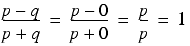The remaining states that we might observe are those where p is greater than q and q is greater than 0. In these cases, we consider the very last vote cast. If the last vote is for Q , we can treat the penultimate vote as the end of a ballot in which P receives p votes and Q receives q -1 votes, for which we assume that the proposition is true. If, however, it is for P , we treat the penultimate vote as the end of a ballot in which P receives p -1 votes and Q receives q votes, for which we also assume that the proposition is true. Now, the probability that the last vote cast is for Q is equal to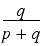and the probability that it is for P is equal to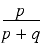Since these two scenarios are independent of each other, the probability that P stays ahead of Q throughout the vote counting is given by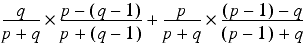Rearranging this, we have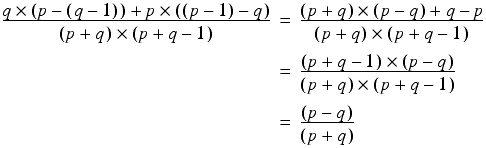We can treat the shorter ballots in exactly the same fashion and must therefore eventually end up with an expression involving a set of ballots in which either the number of votes for P is equal to the number of votes for Q or the number of votes for Q is equal to 0. Hence the proposition must be true whenever p >0 and p q , as claimed. Derivation 1

## What exactly does this have to do with the Countdown numbers game?

Well, er, not very much to be perfectly honest.

That said, the fact that the total number of formulae that can be expressed with up to n distinct variables and the 4 binary operators of addition, subtraction, multiplication and division is equal to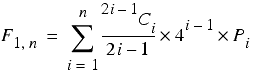is a pretty damn interesting result and, in my opinion at least, was worth a short detour.

Still, I suppose we should probably crack on.

## Implementing RPN operators

The first tool we shall need to assist us in exploring the statistical properties of the Countdown numbers game is a way to transform formula templates into formulae.

We shall begin with an abstract base class to represent an arbitrary RPN operator, called rpn_operator , as illustrated in listing 3.

 ``` template class rpn_operator { public: typedef std::stack > stack_type; virtual ~rpn_operator(); virtual bool apply(stack_type &stack) const = 0; }; template rpn_operator::~rpn_operator() { } ``` Listing 3

Note that we have defined this as a template class. In general, an RPN calculator would operate upon double s, but for the Countdown numbers game we shall need to restrict ourselves to integers. We use the standard stack class for the RPN stack since it does everything we need of it, albeit favouring the processing efficiency of std::vector to the memory efficiency of std::deque .

The return value of the apply member function serves to indicate whether the operation yields a valid result for the given arguments. For the integer only numbers game, we should expect at least half of all division operations to yield a fraction and hence be invalid, since if one argument wholly divides, but is not equal to, the other then the latter cannot wholly divide the former. This is a little too common an outcome to be considered truly exceptional, and so we shall be well advised to avoid the relatively expensive C++ exception mechanism.

The declarations and definitions for our RPN operators are given in listing4. Note that whilst the base class can represent any RPN operator, we are restricting ourselves to the four binary operators used in the numbers game; namely addition, subtraction, multiplication and division.

 ``` template class rpn_add : public rpn_operator { public: virtual bool apply(stack_type &stack) const; }; template class rpn_subtract : public rpn_operator { public: virtual bool apply(stack_type &stack) const; }; template class rpn_multiply : public rpn_operator { public: virtual bool apply(stack_type &stack) const; }; template class rpn_divide : public rpn_operator { public: virtual bool apply(stack_type &stack) const; }; ``` Listing 4

The definitions of the apply member functions are given in listing 5.

 ``` template inline bool rpn_add::apply(stack_type &stack) const { if(stack.size()<2) throw std::invalid_argument(""); const T x0 = stack.top(); stack.pop(); const T x1 = stack.top(); stack.pop(); stack.push(x0+x1); return true; }; template inline bool rpn_subtract::apply(stack_type &stack) const { if(stack.size()<2) throw std::invalid_argument(""); const T x0 = stack.top(); stack.pop(); const T x1 = stack.top(); stack.pop(); stack.push(x0-x1); return true; }; template inline bool rpn_multiply::apply(stack_type &stack) const { if(stack.size()<2) throw std::invalid_argument(""); const T x0 = stack.top(); stack.pop(); const T x1 = stack.top(); stack.pop(); stack.push(x0*x1); return true; }; template inline bool rpn_divide::apply(stack_type &stack) const { if(stack.size()<2) throw std::invalid_argument(""); const T x0 = stack.top(); stack.pop(); const T x1 = stack.top(); stack.pop(); if(!rpn_valid_divide(x0, x1)) return false; stack.push(x0/x1); return true; }; ``` Listing 5

The definitions are pretty straightforward; each operator first checks that there are enough arguments on the stack, then pops them and pushes the result back on to the stack. The only complexity is the call to the as yet undefined rpn_valid_divide function from rpn_divide::apply , which serves to check that the division operation has a valid result.

Illustrated in listing 6, the two overloads distinguish between floating point and integer division. Both return false upon division by zero, with latter additionally checking that the second argument wholly divides the first, since this would be an invalid step in the integer-only numbers game.

 ``` bool rpn_valid_divide(double x0, double x1) { return x1!=0.0; } bool rpn_valid_divide(long x0, long x1) { return x1!=0 && (x0%x1)==0; } ``` Listing 6

Of course, we could do a more thorough job with some template wizardry, but these two overloads will suffice for now.

To be perfectly honest, we should probably perform similar tests for numerical overflow and the like during the application of the operators, perhaps returning an error code instead of a boolean so that we might be able to identify exactly what caused a calculation to fail. That said, these sorts of errors are artefacts of the mechanics of computer arithmetic rather than fundamental limitations of arithmetic and as such are of lesser interest.

## Evaluating formula templates

All that remains to do before we can evaluate our formula templates is to implement a function that does exactly that. This function needs to parse the formula templates, pushing arguments on to the stack or applying operators as required.

The first thing we need is a return type that can indicate both the validity of the result and its value, as given in listing 7.

 ``` template struct rpn_result { rpn_result(); explicit rpn_result(const T &t); bool valid; T value; }; template rpn_result::rpn_result() : valid(false) { } template rpn_result::rpn_result(const T &t) : valid(true), value(t) { } ``` Listing 7

If we have a value with which to construct the result it is, by definition, valid and if not it is, by definition, invalid.

Listing 8 provides the definition of the rpn_evaluate function with which we shall evaluate our formula template. This function takes a formula template, represented by a string containing x and o characters, a vector of pointers to rpn_operator objects and a vector of argument values and returns an rpn_result .

 ``` template rpn_result rpn_evaluate(const std::string &formula, const std::vector const *> &operators, const std::vector &arguments) { typedef rpn_result result_type; typedef typename rpn_operator:: stack_type stack_type; typedef std::vector const *> operators_type; typedef std::vector arguments_type; std::string::const_iterator first_term = formula.begin(); std::string::const_iterator last_term = formula.end(); operators_type::const_iterator first_op = operators.begin(); operators_type::const_iterator last_op = operators.end(); arguments_type::const_iterator first_arg = arguments.begin(); arguments_type::const_iterator last_arg = arguments.end(); stack_type stack; while(first_term!=last_term) { switch(*first_term++) { case 'x': if(first_arg==last_arg) throw std::invalid_argument(""); stack.push(*first_arg++); break; case 'o': if(first_op==last_op) throw std::invalid_argument(""); if(!(*first_op++)->apply(stack)) return result_type(); break; default: throw std::invalid_argument(""); } } if(stack.size()!=1 || first_op!=last_op || first_arg!=last_arg) { throw std::invalid_argument(""); } return result_type(stack.top()); } ``` Listing 8

This function simply iterates over the formula template, pushing the current argument on to the stack of the current term is an x , and applying the current operator if it is an o .

Note that we return an invalid rpn_result in the event that any of the intermediate values in the calculation of the formula are invalid.

If there aren't enough arguments or operators, or if there is not exactly 1 value on the stack at the end of the calculation, the function throws an exception. Recall that the operators themselves throw exceptions if the stack doesn't contain enough arguments when they are applied.

Listing 9 illustrates how we might use rpn_evaluate to calculate the formula 4 2 + 5

 ``` rpn_add add; rpn_multiply multiply; std::vector const *> ops; ops.push_back(&add); ops.push_back(&multiply); std::vector args; args.push_back(4); args.push_back(2); args.push_back(5); std::cout << rpn_evaluate("xxoxo", ops, args).value << std::endl; std::cout << std::endl; ``` Listing 9

If we execute this code, we shall see that the output is 30, as it should be.

Because of the separation of the formula template, the operators and the arguments, the rpn_evaluate function isn't particularly amenable for use as a general purpose calculator. It is, however, very well suited to iterating through the set of all possible formulae so that we can examine the statistical properties of their results.

## Analysing the Countdown numbers game

We are now very nearly ready to investigate the statistical properties of the numbers game. All that is left for us to do is to work out how to iterate through every possible set of operators and arguments that might be substituted into the formula templates generated by our all_templates function.

We have a clue as to how to go about this in the formula we derived for calculating the total number of possible formulae. Firstly, we shall need a mechanism for enumerating the 24 C 6 combinations of 6 numbers from the 24 available. For each of these combinations we shall then need to iterate over the formula templates, setting n to the number of arguments that each in turn requires and enumerating for them the 4n-1 sets of operators and the 6 P n permutations of arguments.

We shall take as our inspiration the standard next_permutation function with which we can iterate through the set of full permutations of the elements in a given iterator range.

We shall, however, have to wait until next time to transform inspiration into computation, since I have regrettably run out of space in this instalment. So until then, dear reader, do take my advice and, if at all possible, try to catch an episode or two of that most regal of the tea-time quiz fraternity; my beloved Countdown.

## Acknowledgements

[Countdown] http://www.channel4.com/programmes/countdown

[Feller68] Feller, W., An Introduction to Probability Theory and its Applications, vol. 1, 3rd ed., Wiley, 1968.

[Renault07] Renault, M., 'Four Proofs of the Ballot Theorem', Mathematics Magazine, vol. 80, pp. 345-352, 2007.